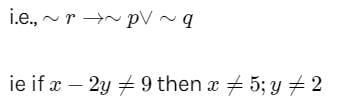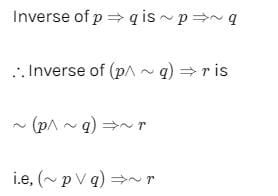Test: Mathematical Reasoning- 1

# Test: Mathematical Reasoning- 1

Test Description

## 25 Questions MCQ Test Mathematics (Maths) Class 11 | Test: Mathematical Reasoning- 1

Test: Mathematical Reasoning- 1 for Commerce 2023 is part of Mathematics (Maths) Class 11 preparation. The Test: Mathematical Reasoning- 1 questions and answers have been prepared according to the Commerce exam syllabus.The Test: Mathematical Reasoning- 1 MCQs are made for Commerce 2023 Exam. Find important definitions, questions, notes, meanings, examples, exercises, MCQs and online tests for Test: Mathematical Reasoning- 1 below.
Solutions of Test: Mathematical Reasoning- 1 questions in English are available as part of our Mathematics (Maths) Class 11 for Commerce & Test: Mathematical Reasoning- 1 solutions in Hindi for Mathematics (Maths) Class 11 course. Download more important topics, notes, lectures and mock test series for Commerce Exam by signing up for free. Attempt Test: Mathematical Reasoning- 1 | 25 questions in 25 minutes | Mock test for Commerce preparation | Free important questions MCQ to study Mathematics (Maths) Class 11 for Commerce Exam | Download free PDF with solutions
 1 Crore+ students have signed up on EduRev. Have you?
Test: Mathematical Reasoning- 1 - Question 1

### Which of the following is a preposition?

Test: Mathematical Reasoning- 1 - Question 2

### Let p and q be two propositions given by P : The sky is blue, q : Milk is white, Then , p^q is

Detailed Solution for Test: Mathematical Reasoning- 1 - Question 2

p∧q means statement p and q

=> The sky is blue and  milk is white.

Test: Mathematical Reasoning- 1 - Question 3

### Let p and q be two prepositions given by p : I play cricket during the holidays, q : I just sleep throughout the day then , the compound statement p ∧ q is

Detailed Solution for Test: Mathematical Reasoning- 1 - Question 3

∧ is replaced by and in verbal form

Test: Mathematical Reasoning- 1 - Question 4

Let p and q be two prepositions given by p : It is hot, q : He wants water Then , the verbal meaning of p → q is

Test: Mathematical Reasoning- 1 - Question 5

Let p and q be two prepositions given by p : I take medicine, q : I can sleep then ,the compound statement ∼p→∼q means

Test: Mathematical Reasoning- 1 - Question 6

Which of the following is true for the propositions p and q ?

Test: Mathematical Reasoning- 1 - Question 7

Which of the following is a compound statement

Detailed Solution for Test: Mathematical Reasoning- 1 - Question 7

Compound statement is connected with And , or , etc.
So, the statement 7 is both odd and prime number is a compound statement.

Test: Mathematical Reasoning- 1 - Question 8

Which of the following is always true ?

Test: Mathematical Reasoning- 1 - Question 9

Negation of the statement

∼p→(q∨r)∼p→(q∨r) is

Test: Mathematical Reasoning- 1 - Question 10

For any three propositions p, q, and r, the proposition (p∧q)∧(q∧r) is true, when

Test: Mathematical Reasoning- 1 - Question 11

The negation of the proposition “if a quadrilateral is a square, then it is a rhombus “ is

Test: Mathematical Reasoning- 1 - Question 12

The contrapositive of the statement “ if 22 = 5, then I get first class” is

Test: Mathematical Reasoning- 1 - Question 13

If x = 5 and y = - 2, then x – 2y = 9. The contrapositive of this proposition is

Detailed Solution for Test: Mathematical Reasoning- 1 - Question 13

Let p,q and r be three propositions given by
p:x=5; q:y=−2 and r:x−2y=9
Then, the given statement is (p∧q)→r
Its contrapositive isTest: Mathematical Reasoning- 1 - Question 14

Let p and q be two propositions. Then, the contrapositive of the implication p→q is

Test: Mathematical Reasoning- 1 - Question 15

Which of the following statement is a tautology ?

Test: Mathematical Reasoning- 1 - Question 16

The statement p→(q→p) is equivalent to

Test: Mathematical Reasoning- 1 - Question 17

The inverse of the proposition (p∧∼q)→ r is

Detailed Solution for Test: Mathematical Reasoning- 1 - Question 17Test: Mathematical Reasoning- 1 - Question 18

Logical equivalent proposition to the proposition ∼(p∨q) is

*Multiple options can be correct
Test: Mathematical Reasoning- 1 - Question 19

Let p and q be two propositions. Then the implication p↔∼q is true ,when

Detailed Solution for Test: Mathematical Reasoning- 1 - Question 19

Explanation:- (p↔∼q) will be true

if both p and ∼q are true ⟹p is true and q is false

Or if both p and ∼q are true ⟹p is false and q is true

Test: Mathematical Reasoning- 1 - Question 20

Which of the following is a proposition ?

Test: Mathematical Reasoning- 1 - Question 21

The contrapositive of 2x + 3 = 9 ⇒ x ≠ 4 is

Test: Mathematical Reasoning- 1 - Question 22

Consider the proposition: “If we control population growth, we prosper “. Negative of this proposition is

Test: Mathematical Reasoning- 1 - Question 23

Consider the proposition: “If the pressure increases, the volume decreases “. Negative of this proposition is

Test: Mathematical Reasoning- 1 - Question 24

Which of the following connectives satisfy commutative law ?

Test: Mathematical Reasoning- 1 - Question 25

Which of the following pairs is logically equivalent ?

## Mathematics (Maths) Class 11

156 videos|176 docs|132 tests
 Use Code STAYHOME200 and get INR 200 additional OFF Use Coupon Code
Information about Test: Mathematical Reasoning- 1 Page
In this test you can find the Exam questions for Test: Mathematical Reasoning- 1 solved & explained in the simplest way possible. Besides giving Questions and answers for Test: Mathematical Reasoning- 1, EduRev gives you an ample number of Online tests for practice

## Mathematics (Maths) Class 11

156 videos|176 docs|132 tests